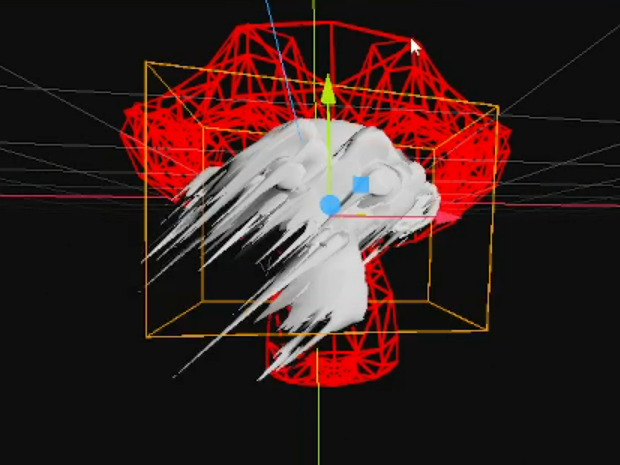# Mesh smearing

This tweet has been making the rounds lately:

It shows a Blender shader that stretches parts of a mesh based on it’s motion. I would like to share my approach to recreating the effect inside of Godot 4.

Before reading on, I encourage you to try do it yourself without any hints!

• Define an additional transform which lags behind the current object, let’s call it the `lag_transform`
• Interpolate between the transforms in the vertex shader

This way, it works naturally with any affine transformation (e.g. translation, rotation, scale). A parameter, `t`, determines exactly where the vertices land between their original position and the lagged position. As this happens on a per-vertex basis, noise can then be used to modulate the displacement over the surface of the mesh.

See the final code below

GDScript

``````@tool
extends GeometryInstance3D

@export_range(0.0,16.0) var weight := 8.0

func _process(delta):
# lag_transform gradually approaches the current transform over time
lag_transform = lag_transform.interpolate_with(global_transform, weight*delta)

``````
``````shader_type spatial;

uniform sampler2D noise;
uniform mat4 lag_transform;

void vertex(){
// a parameter determining magnitude of the displacement
float t = pow(texture(noise, UV * 5.0).x, 6.5) * 2.0;

// linearly interpolate between the current transform and the laggy transform in world space
MODELVIEW_MATRIX = MODEL_MATRIX + (lag_transform - MODEL_MATRIX)*t;

// puts it into view space
MODELVIEW_MATRIX = VIEW_MATRIX * MODELVIEW_MATRIX;
}``````
###### Tags
displacement, smear, smear frame, stylizedThe shader code and all code snippets in this post are under MIT license and can be used freely. Images and videos, and assets depicted in those, do not fall under this license. For more info, see our License terms.

## More from celyk

### UV and Normals Unwrap (Mesh Projection as UV) World Aligned

Subscribe
Notify of